Ex 4.5

Chapter 4 Class 12 Determinants
Serial order wise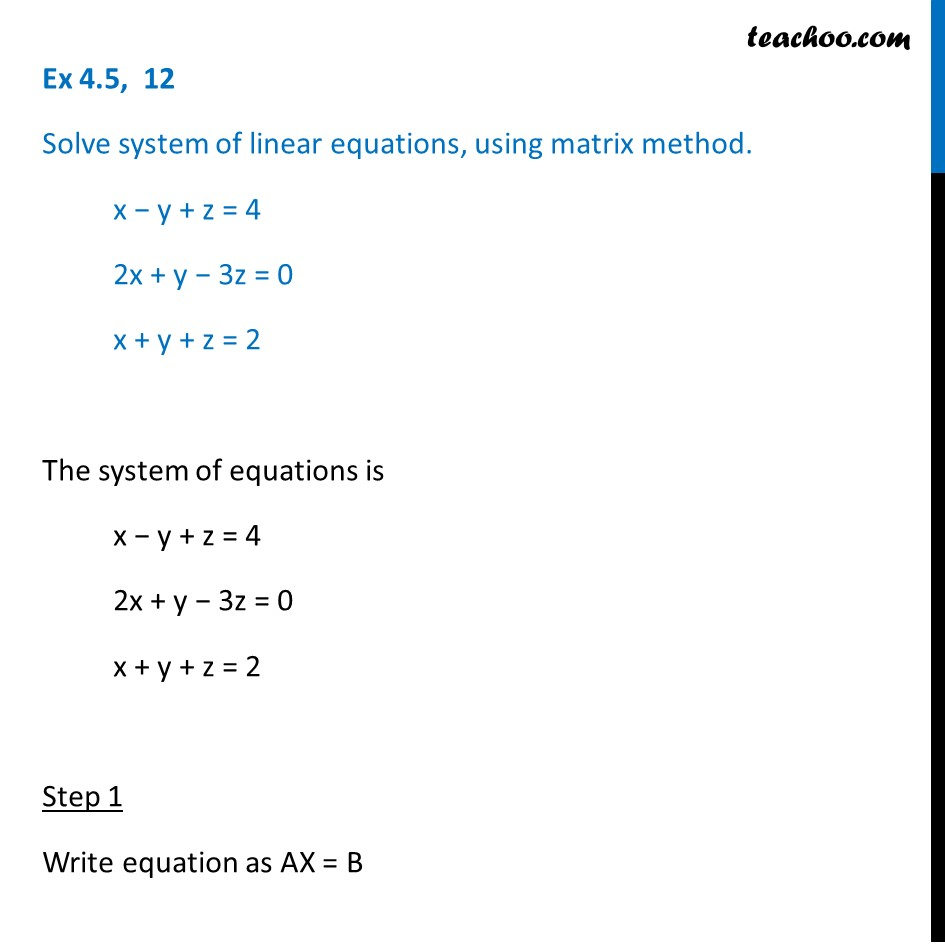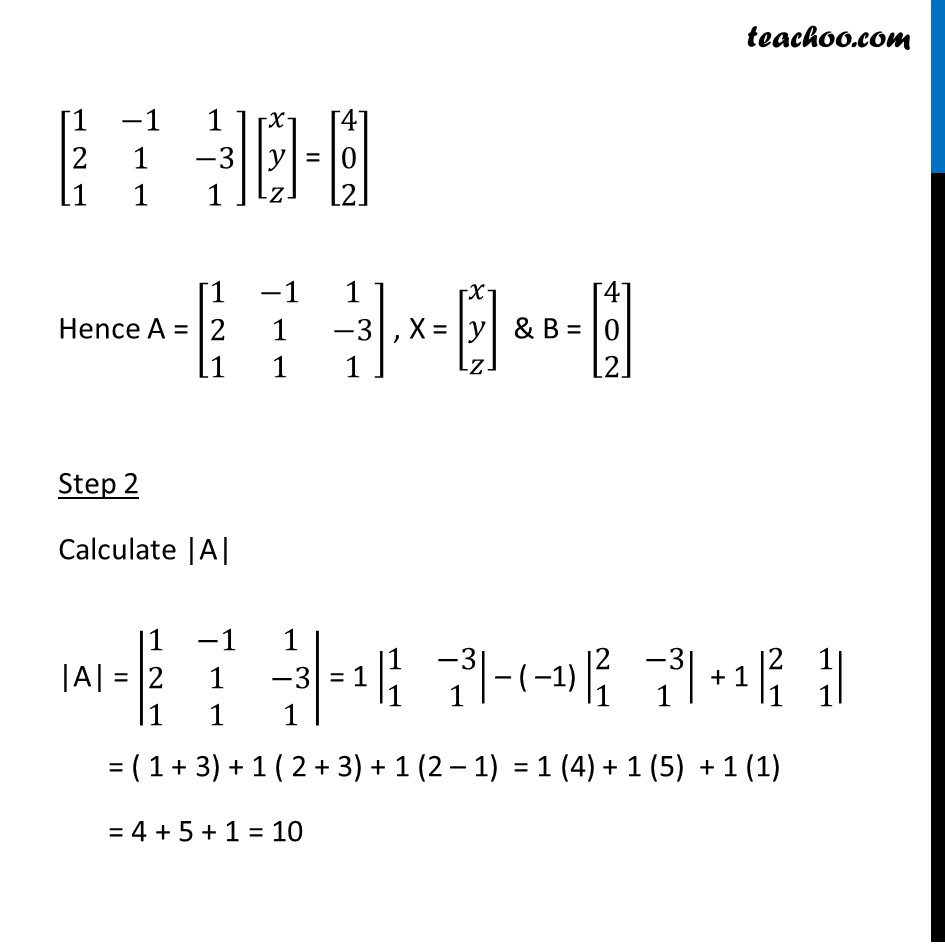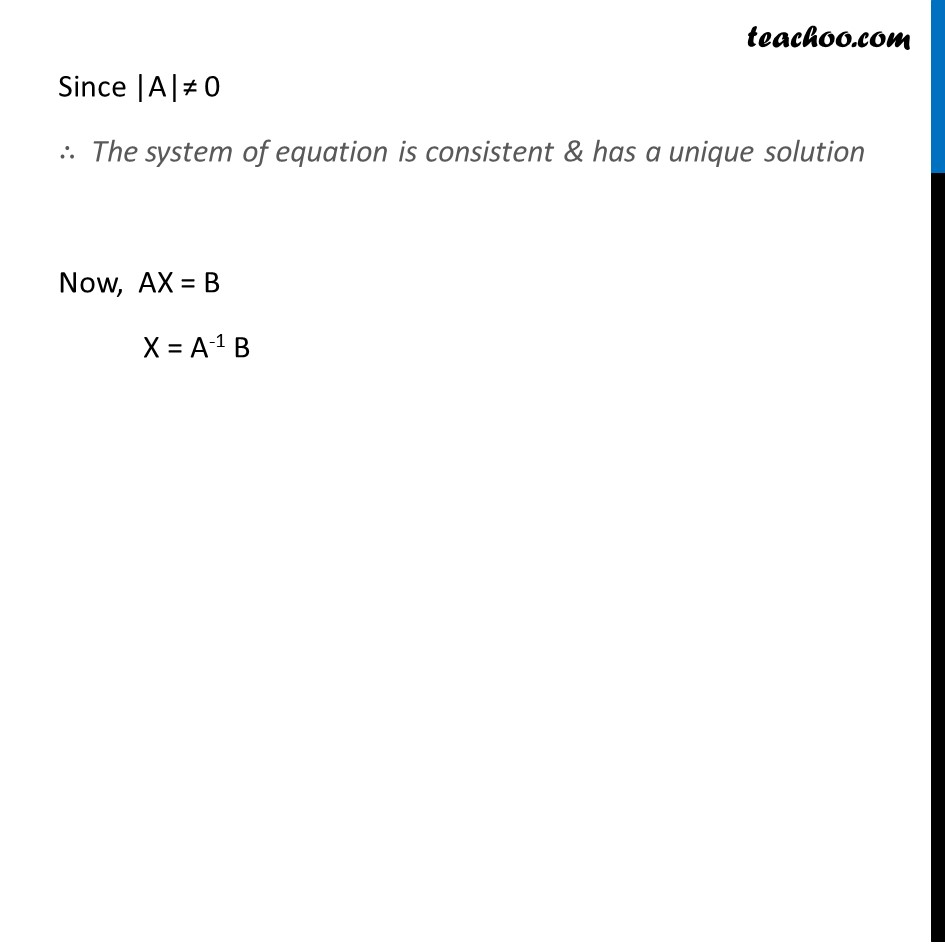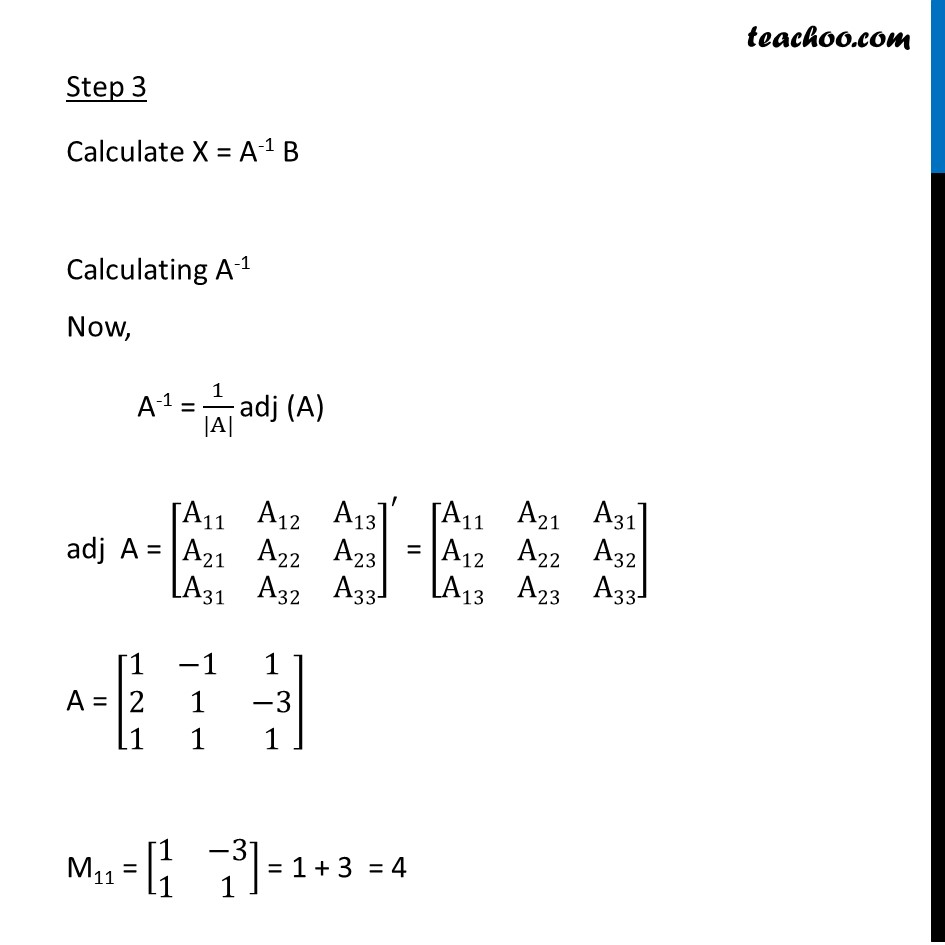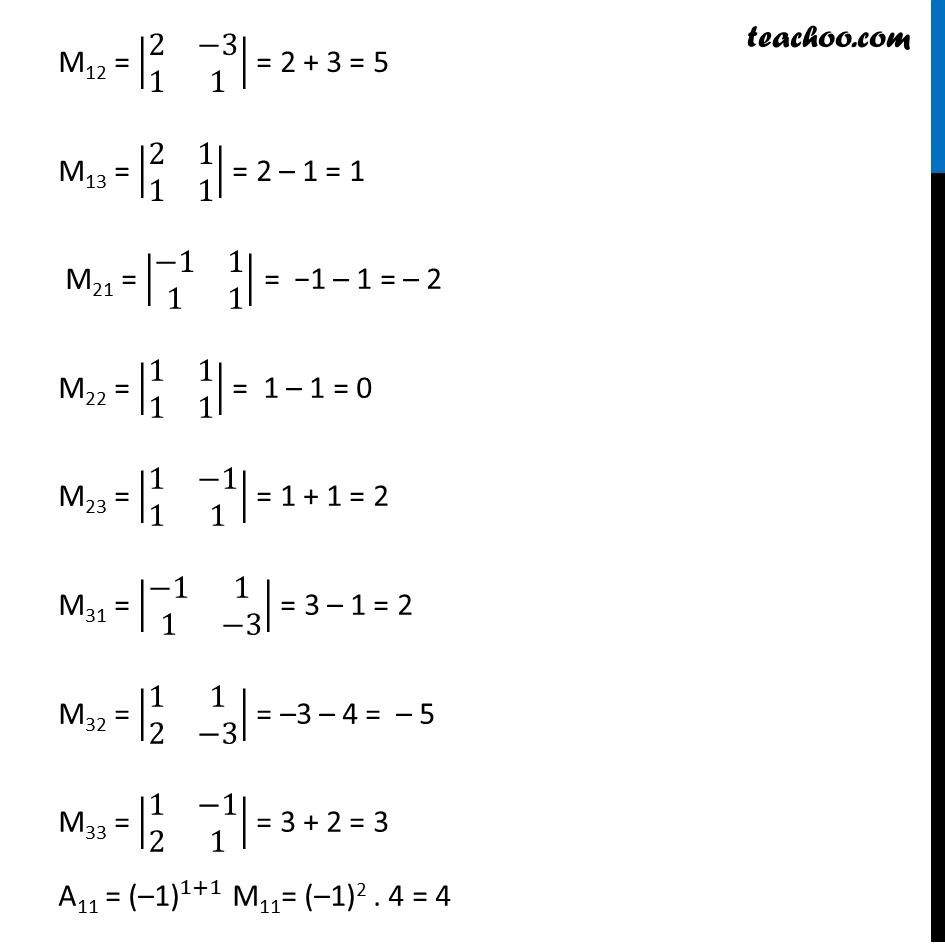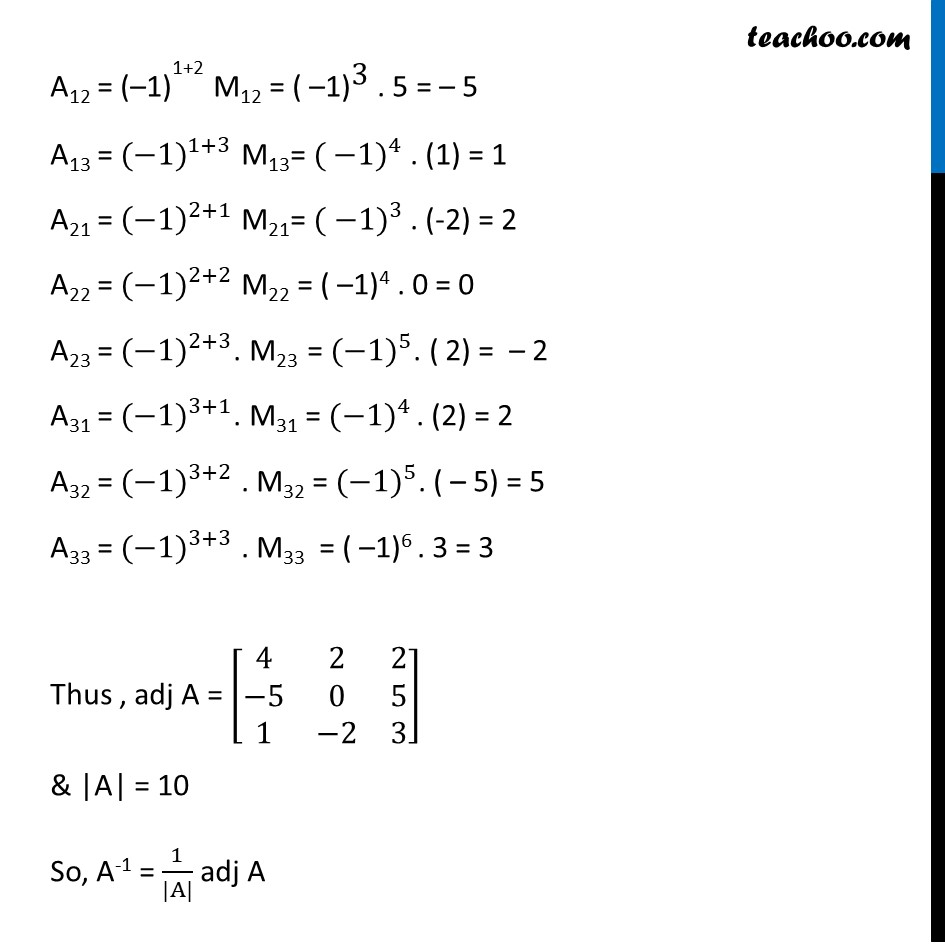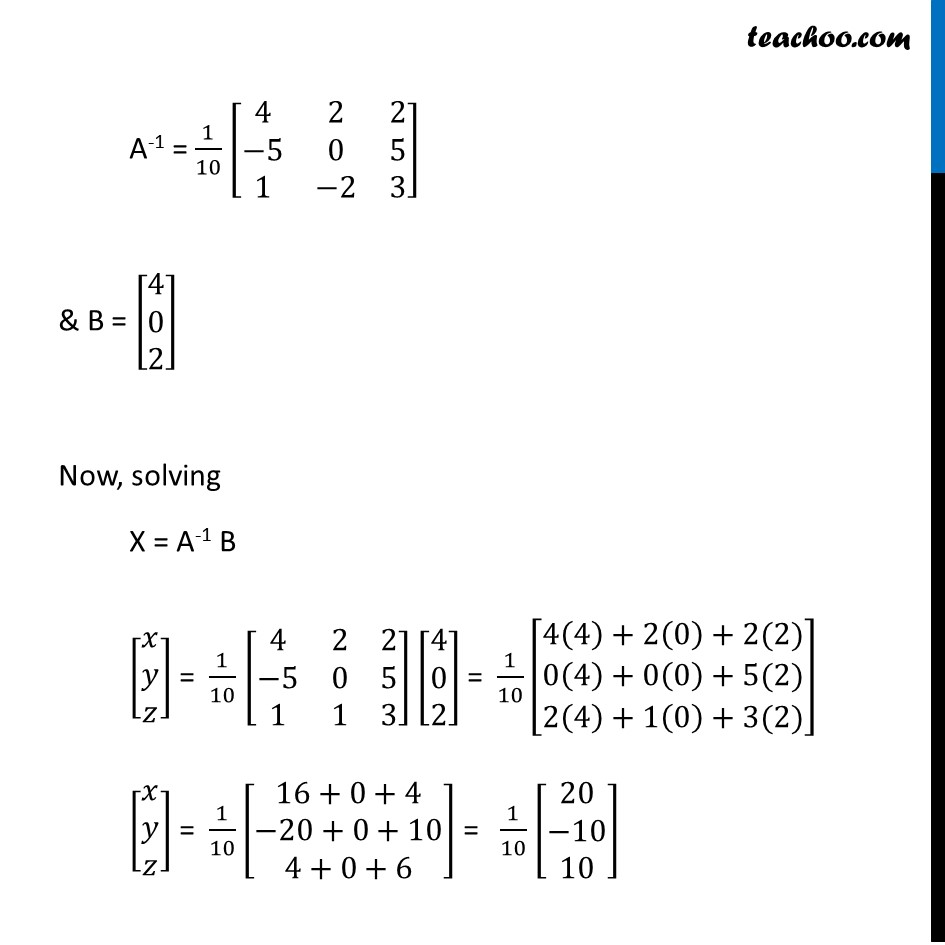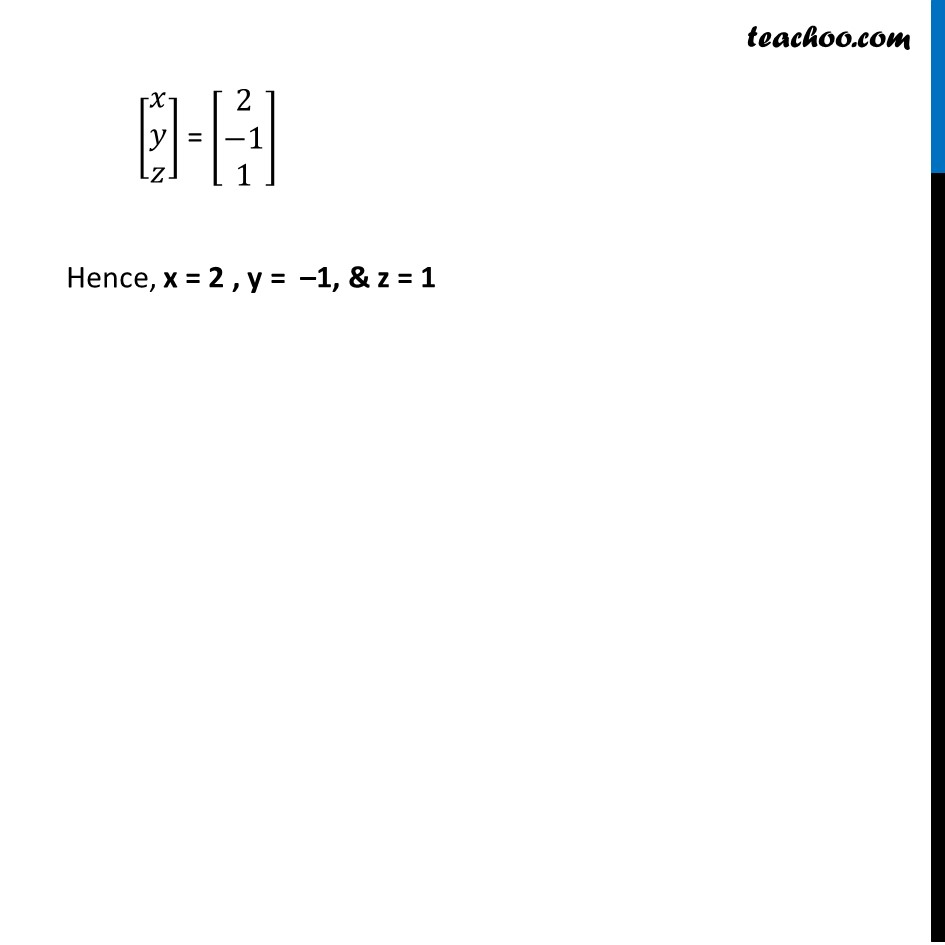Learn in your speed, with individual attention - Teachoo Maths 1-on-1 Class

### Transcript

Ex 4.5, 12 Solve system of linear equations, using matrix method. x − y + z = 4 2x + y − 3z = 0 x + y + z = 2 The system of equations is x − y + z = 4 2x + y − 3z = 0 x + y + z = 2 Step 1 Write equation as AX = B [■8(1&−1&1@2&1&−3@1&1&1)] [■8(𝑥@𝑦@𝑧)] = [■8(4@0@2)] Hence A = [■8(1&−1&1@2&1&−3@1&1&1)] , X = [■8(𝑥@𝑦@𝑧)] & B = [■8(4@0@2)] Step 2 Calculate |A| |A| = |■8(1&−1&1@2&1&−3@1&1&1)| = 1 |■8(1&−3@1&1)| – ( –1) |■8(2&−3@1&1)| + 1 |■8(2&1@1&1)| = ( 1 + 3) + 1 ( 2 + 3) + 1 (2 – 1) = 1 (4) + 1 (5) + 1 (1) = 4 + 5 + 1 = 10 Since |A|≠ 0 ∴ The system of equation is consistent & has a unique solution Now, AX = B X = A-1 B Step 3 Calculate X = A-1 B Calculating A-1 Now, A-1 = 1/(|A|) adj (A) adj A = [■8(A11&A12&A13@A21&A22&A23@A31&A32&A33)]^′ = [■8(A11&A21&A31@A12&A22&A32@A13&A23&A33)] A = [■8(1&−1&1@2&1&−3@1&1&1)] M11 = [■8(1&−3@1&1)] = 1 + 3 = 4 M12 = |■8(2&−3@1&1)| = 2 + 3 = 5 M13 = |■8(2&1@1&1)| = 2 – 1 = 1 M21 = |■8(−1&1@1&1)| = −1 – 1 = – 2 M22 = |■8(1&1@1&1)| = 1 – 1 = 0 M23 = |■8(1&−1@1&1)| = 1 + 1 = 2 M31 = |■8(−1&1@1&−3)| = 3 – 1 = 2 M32 = |■8(1&1@2&−3)| = –3 – 4 = – 5 M33 = |■8(1&−1@2&1)| = 3 + 2 = 3 A11 = 〖"(–1)" 〗^(1+1) M11= (–1)2 . 4 = 4 A12 = 〖"(–1)" 〗^"1+2" M12 = 〖"( –1)" 〗^"3" . 5 = – 5 A13 = 〖(−1)〗^(1+3) M13= 〖( −1)〗^4 . (1) = 1 A21 = (−1)^(2+1) M21= 〖( −1)〗^3 . (-2) = 2 A22 = 〖(−1)〗^(2+2) M22 = ( –1)4 . 0 = 0 A23 = 〖(−1)〗^(2+3). M23 = 〖(−1)〗^5. ( 2) = – 2 A31 = 〖(−1)〗^(3+1). M31 = 〖(−1)〗^4 . (2) = 2 A32 = 〖(−1)〗^(3+2) . M32 = 〖(−1)〗^5. ( – 5) = 5 A33 = 〖(−1)〗^(3+3) . M33 = ( –1)6 . 3 = 3 Thus , adj A = [■8(4&2&2@−5&0&5@1&−2&3)] & |A| = 10 So, A-1 = 1/(|A|) adj A A-1 = 1/10 [■8(4&2&2@−5&0&5@1&−2&3)] & B = [■8(4@0@2)] Now, solving X = A-1 B [■8(𝑥@𝑦@𝑧)] = 1/10 [■8(4&2&2@−5&0&5@1&1&3)] [■8(4@0@2)] = 1/10 [■8(4(4)+2(0)+2(2)@0(4)+0(0)+5(2)@2(4)+1(0)+3(2))] [■8(𝑥@𝑦@𝑧)] = 1/10 [■8(16+0+4@−20+0+10@4+0+6)] = 1/10 [■8(20@−10@10)] [■8(𝑥@𝑦@𝑧)] = [■8(2@−1@1)] Hence, x = 2 , y = –1, & z = 1